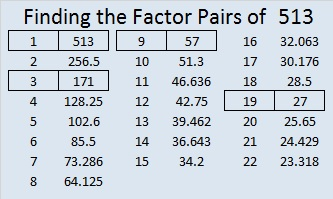# Reducible Square Roots Up to √513

5 + 1 + 3 = 9 so 513 can be evenly divided by 9, and thus its square root can be simplified.

513 is the 200th counting number whose square root can be reduced. 200/513 ≈ .38986, which means, so far, 38.97% of the counting numbers have reducible square roots.

Here are the first 100 reducible square roots followed by the second hundred:I highlighted the ones that are part of three or more consecutive reducible square roots.• 513 is a composite number.
• Prime factorization: 513 = 3 x 3 x 3 x 19, which can be written 513 = (3^3) x 19
• The exponents in the prime factorization are 3 and 1. Adding one to each and multiplying we get (3 + 1)(1 + 1) = 4 x 2 = 8. Therefore 513 has exactly 8 factors.
• Factors of 513: 1, 3, 9, 19, 27, 57, 171, 513
• Factor pairs: 513 = 1 x 513, 3 x 171, 9 x 57, or 19 x 27
• Taking the factor pair with the largest square number factor, we get √513 = (√9)(√57) = 3√57 ≈ 22.64950331.Paula Beardell Krieg
•ivasallay